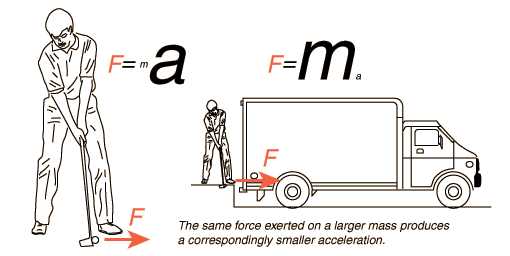## 1- Forces and Motion

Force. (vector)

A force is a push or a pull, exerted by one object on another.

In SI force measured in or kg.m/s2

It has a direction and a magnitude so it is vector

Force (F) = mass (m) x acceleration (a)

Force depends mass and

• Acceleration is directly proportional to force.
• Acceleration is inversely proportional to mass.

The results of the same force exerted on objects of different mass.